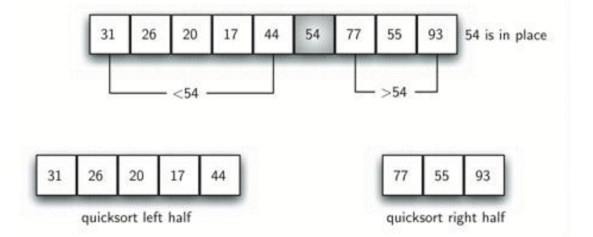# Quick Sort

The quick sort uses divide and conquer to gain the same advantages as the merge sort, while not using additional storage.

Quick Sort

The quick sort uses divide and conquer to gain the same advantages as the merge sort, while not using additional storage. As a trade-off, however, it is possible that the list may not be divided in half. When this happens, we will see that performance is diminished. A quick sort first selects a value, which is called the pivot value. Although there are many different ways to choose the pivot value, we will simply use the first item in the list. The role of the pivot value is to assist with splitting the list. The actual position where the pivot value belongs in the final sorted list, commonly called the split point, will be used to divide the list for subsequent calls to the quick sort. Figure shows that 54 will serve as our first pivot value. Since we have looked at this example a few times already, we know that 54 will eventually end up in the position currently holding 31.The partitionprocess will happen next. It will find the split point and at the same time move other items to the appropriate side of the list, either less than or greater than the pivot value. Partitioning begins by locating two position markers—let’s call them leftmark and rightmark—at the beginning and end of the remaining items in the list (positions 1 and 8 in Figure below. The goal of the partition process is to move items that are on the wrong side with respect to the pivot value while also converging on the split point. Figure above shows this process as we locate the position of 54.

We begin by incrementing leftmark until we locate a value that is greater than the pivot value. We then decrement rightmark until we find a value that is less than the pivot value. At this point we have discovered two items that are out of place with respect to the eventual split point. For our example, this occurs at 93 and 20. Now we can exchange these two items and then repeat the process again. At the point where rightmark becomes less than leftmark, we stop. The position of rightmark is now the split point. The pivot value can be exchanged with the contents of the split point and the pivot value is now in place. In addition, all the items to the left of the split point are less than the pivot value, and all the items to the right of the split point are greater than the pivot value. The list can now be divided at the split point and the quick sort can be invoked recursively on the two halves.The quickSort function shown in CodeLens 7 invokes a recursive function, quickSortHelper.quickSortHelper begins with the same base case as the merge sort. If the length of the list is less than or equal to one, it is already sorted. If it is greater, then it can be partitioned and recursively sorted. Thepartition function implements the process described earlier.

QUICK SORT for PSEUDOCODE:

def quickSort(alist):

quickSortHelper(alist,0,len(alist)-1)

def quickSortHelper(alist,first,last): if first<last:

splitpoint = partition(alist,first,last)

quickSortHelper(alist,first,splitpoint-1)

quickSortHelper(alist,splitpoint+1,last)

def partition(alist,first,last):

pivotvalue = alist[first]

leftmark = first+1

rightmark = last

done = False

while not done:

while leftmark <= rightmark and \

alist[leftmark] <= pivotvalue: leftmark = leftmark + 1

while alist[rightmark] >= pivotvalue and \ rightmark >= leftmark:

rightmark = rightmark -1 if rightmark < leftmark: done = True

else:

temp = alist[leftmark]

alist[leftmark] = alist[rightmark]

alist[rightmark] = temp

temp = alist[first]

alist[first] = alist[rightmark]

alist[rightmark] = temp

return rightmark

alist = [54,26,93,17,77,31,44,55,20]

quickSort(alist)

print(alist)

Study Material, Lecturing Notes, Assignment, Reference, Wiki description explanation, brief detail
Object Oriented Programming and Data Structure : Sorting And Searching : Quick Sort |